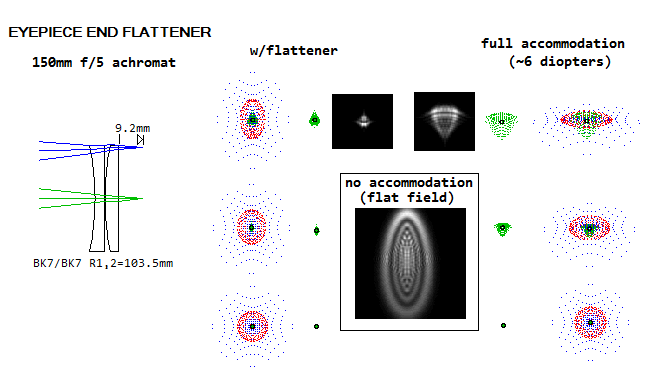telescopeѲptics.net          ▪▪▪▪                                             CONTENTS

# 10.1.2. Sub-aperture corrector: Field-flattener

Image field curvature is not a serious detriment to visual observing, due to the natural ability of the eye to accommodate (refocus). In photographic use, however, field curvature induces defocus error, which can be significant. In order to flatten curved image field, an auxiliary lens, or group of lens is placed in front of the final focus, offsetting image curvature by generating one of similar magnitude but opposite in sign. In general, a positive lens, or group of lenses, generates curvature concave towards it, while a negative lens generates image curvature convex toward it.

Single-lens flattener

The simplest way to correct for field curvature is by placing a single thin lens just in front of the final focus. With proper choice of parameters, it flattens the field while inducing very low aberrations, except at very large relative apertures. Assuming no appreciable astigmatism induced by the lens itself - valid for telescopes, in general, but not for the Schmidt camera, or other very fast systems - the field is flattened when lens' Petzval curvature is equal, and opposite in sign, to the median field curvature of a telescope. This determines needed lens shape as plano-concave (negative) for for Cassegrain-like telescopes, and plano-convex (positive) for the Gregorian.

By default, field flattener lens faces image with its flat side, and the sign of its curved surface is determined by the sign of image curvature. Needed radius of curvature of the lens is: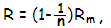with n being the lens refractive, and Rm the telescope median (best astigmatic) image radius. This, of course, assumes that the lens itself generates zero astigmatism; if it is not so, the needed radius is modified according to the magnitude and sign of lens astigmatism.

For the Schmidt camera, field flattener lens is positive, with the radius R=[1-(1/n)]RM/2, with RM being the mirror radius of curvature (since it has no astigmatism in the Schmidt arrangement, its best surface coincides with its Petzval surface, which equals RM/2).

For the Newtonian, field flattener lens is of negative power, with the radius R=[1-(1/n)]Rm/2 (according to the sign convention, with the best field concave toward mirror, Rm is numerically positive in the Newtonian), and so is for the refractor. For the latter, median field curvature varies from one to another type; for a typical doublet achromat median field curvature is approximately -ƒ/3, ƒ being the refractor focal length, and the field flattener's radius R~-[1-(1/n)]ƒ/3 (of negative power).

EXAMPLE 1: Schmidt camera field flattener -  Good example for the use of a single-lens flattener is the Schmidt camera, which is exclusively a photographic instrument. Its only remaining primary aberration is strong field curvature. This leaves two choices: either use of a curved detector, or sacrificing much of the exquisite field quality by using flat detector.

In general, lens aberrations are low if it remains very close to the original focus. But even then, they can be significant with wide angular fields and/or fast focal ratios. Due to the lens thickness being significant relative to object and image separation, the thin lens equations are not appropriate. Instead, aberrations are calculated for each lens surface, similarly to the approach described with the generalized aberration coefficients. Only in this case, with the aperture stop displaced from the front lens surface, the relations need to account for this factor.

Numerical value of the stop separation T is determined by system configuration: in the Schmidt camera, for the front lens (flattener's) surface it is the distance from the surface to the corrector (where the mirror re-images the stop), thus numerically negative.

For the second lens surface the stop separation is given by T2=α'ch1, h1 being the chief ray height on the second surface (illustrated below; Schmidt flattener thickness exaggerated to show ray paths). In the Newtonian, T1 it is the distance from the lens' front surface to the mirror, numerically positive (ASM on the illustration below, as opposed to ASS, with the aperture stop at the surface and T1=0). In two-mirror systems, it is the distance from the lens' surface to the image of the aperture stop (primary) formed by the secondary (exit pupil of the system, ExP on the illustration), numerically negative.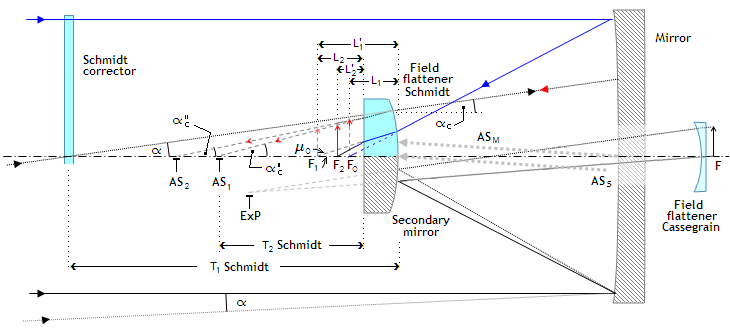Likewise, the chief ray angle αc is determined by system configuration: in the Schmidt camera, the chief ray coming through the center of the corrector is reflected back to the same point, therefore converging toward the front lens surface at the same incident angle α. Since it is opening upward from the axis, it is numerically positive, and so is the projected normal to the surface angle δ at the axis. Since the angles are small enough to be expressed in radians, it determines the chief ray angle α'c after refraction at the front lens surface as α'c=δ-(δ-α)/n, with all the angles in radians, n being the glass refractive. In a two-mirror system, the lens field flattener is of negative power, with both α'cs (chief ray angle after reflection from the secondary, appearing to be coming from the image of the primary formed by the secondary) and the surface normal angle δ numerically negative, the chief ray angle after the front lens surface α'c=δ-(δ-α)/n.

Since the rear surface of the flattener is flat, α'c=nαc. The angle μ1=1/2nF for the marginal ray of axial cone determines location of the image formed by the first lens surface as L1'=h00 from the front lens surface, h0 being the height of marginal ray at the surface, given as h0=l/2F, l being the front lens surface to original focus separation, and F the focal ratio.

This outlines the general procedure for calculating aberrations of a singlet field flattener lens facing focus with its flat side.

With spherical lens surface, Q=0 and the aberration coefficients for spherical aberration, coma and astigmatism, from Eq. (k)-(m), are given by

s=-NJ2/8,  c=NJYh/2  and  a=-N(Yh)2/2, respectively, with

N=n2[(1/n'L')-(1/nL)],     J=[(1/L)-(1/R)],     Y=[(1/T)-(1/R)]

and the height of incident point on the surface h=(Tα), where n, n' are the refractive indici of the incident and refractive/reflecting media, L, L' the surface-to-object and image separation, respectively, R being the lens surface radius of curvature, T being the surface-to-stop separation and α the chief ray angle in radians. Note that the refractive indici are numerically negative, which requires appropriate adjustment to some of the general relations. For instance, needed lens surface curvature to flatten the field is R=(1-1/nl)Rm/2 with the index n numerically positive, and R=(1+1/nl)Rm/2 with the index negative.

With L«R, Eq. 1 gives L'~n'L/n which, after substitution in the relation for N gives N~n(n2-n'2)/(n'2L); also, J~(1/L) for the front lens surface. In terms of the final lens to image separation, L2', L1=L2'+(t/n), L1'=nL2'+t, and L2=nL2'.

Stop separation for the front flattener surface is equal to its separation to the corrector, thus T1=Rm/2, Rm being the mirror radius of curvature, and the chief angle αc for the front surface equals the field angle α. For the rear lens surface, the chief ray angle is, from the ray geometry, αc'=(α'/n)-[1+(1/n)]γ, with γ=αcT1/R being the lens radius of curvature angle at the front surface point of incidence, and the stop separation for the rear surface T2=c/α'c)T1. Alternately, from Eq. 1, T2=n2RT1/[(n2+1)T1-R]. Note that all three angles are numerically positive.

After laying down the formalism, here is the actual example: lower-order aberrations induced by a singlet field flattener lens in 200mm ƒ/2 Schmidt camera (SPECS). For near-minimum lens-image separation of 2mm, lens thickness t=-3mm, lens refractive n'1=n2=nl=-1.5, the image shift caused by the lens is approximately [1+(1/nl)]t=-1mm, which determines lens location such that its rear surface is ~1mm inside the original focus; thus, the front surface to the original focus separation l=-4mm.

At this location, the height of marginal ray at the front lens surface - determining the effective (and minimum) front lens aperture semi-diameter d1 - is d1=-l/2F= 1mm (the converging angle represented by 1/2F is numerically negative, since opening counterclockwise, which is why it requires minus sign when expressed using the F-number). The indici are n1=-1 and n'1=-1.5. The front lens surface stop separation T1=-404mm, and the height of the incidence point at the front surface for the field (and aperture stop) chief ray angle αc=α=2° is h1=cT1=404αc=14.1mm. The object distance L equals the separation between lens surface and the image formed by preceding surface, thus L1=-4mm. The radius of curvature of the front lens surface needed to flatten the field (assuming near zero lens astigmatism) is

R=[1+(1/nl)]Rm/2=-133mm.

For the rear surface, n=-1.5, n'=-1. With L'1=-nlL1=1.5L1, aperture radius d2=[1+(t/nlL1)]d1=0.5mm. Similar result is obtained using the refracted marginal ray angle of the axial cone μ=(1/2nF)+[1+(1/n)]d1/R, from d2=d1-μt=0.49mm. The object distance for this surface is L2=L'1-t=-3mm, and the image distance L2'=-2mm. The chief ray angle at the rear surface α'c=0.058, the stop separation T2=(αc/α'c)T1=-242mm, and the incidence point height for the chief ray h2=h1T2/(T2+t)=13.9.

The corresponding values of compounded parameters for the front lens surface are N1=-0.139, J1=-0.25, Y1=0.005, and h1=14.1, giving the aberration coefficients

s1=0.0011, c1=0.0012, and a1=0.00035.

For the rear surface, N2=0.625, J2=-0.33, Y2=-0.0041 and h2=13.9mm, giving the aberration coefficients

s2=-0.0085, c2=0.0059 and a2=-0.001.

The corresponding combined coefficients for both lens surfaces are

sl=s1+(d2/d1)4s2=0.00057, cl=c1+(d2/d1)3c2=0.0019 and al=a1+(d2/d1)2c2=0.0001,

with the resulting P-V wavefront errors at paraxial focus, in units of 0.00055mm wavelength,

Ws=sld14=-1, Wc=2cld13=6.9 and Wa=2ald12=0.36

for spherical aberration, coma and astigmatism, respectively. At the best focus location, the errors  are smaller by a factor -0.25 for spherical aberration, and 1/3 for coma (P-V for the astigmatism remains unchanged, but the RMS error at the best focus is smaller by a factor of 1/1.5), which gives the final values as

Ws=0.25, Wc=2.3 and Wa=0.36.

The exact raytrace by OSLO gives for this system Ws=0.2 (the differential mainly due to the residual spherical of the camera), Wc=2.36 and Wa=0.09 (the latter two based on the Zernike aberration coefficients for primary aberrations, with the higher order terms negligible) for the lower-order aberrations at the best focus location. Most of the lens' astigmatism - about 3/4 of it - is offset by the corrector's astigmatism of opposite sign, with the effective system astigmatism coming at less than 1/10 wave at 2° off axis. Higher-order aberrations are still low, but increasing rapidly with larger relative apertures. Here is the raytrace plot: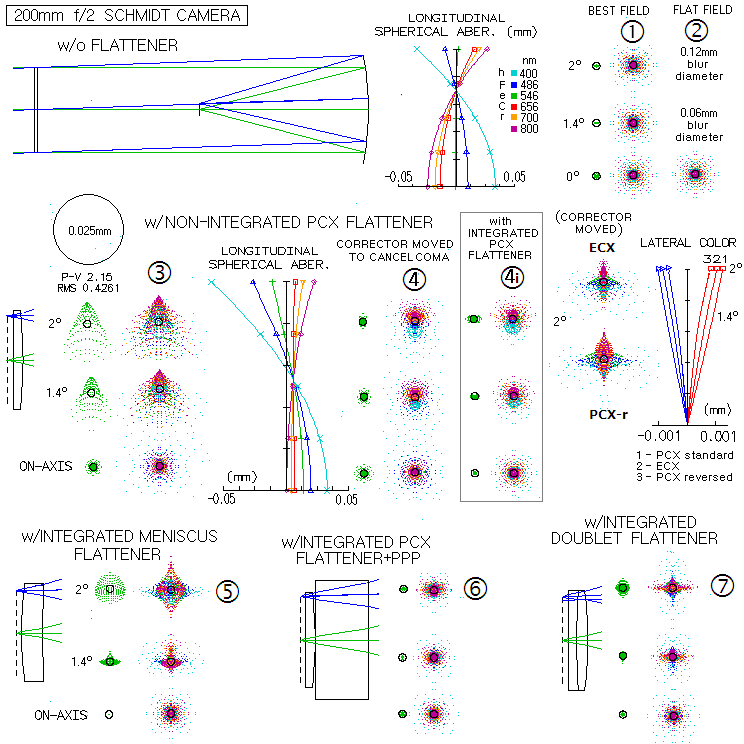Evidently, the most significant aberration induced by the flattener is coma. An option for eliminating this (negative) coma is to move Schmidt corrector somewhat closer to the primary. For this particular system, the separation nearly eliminating coma, with little effect on other aberrations, is 772mm (28mm closer than the original camera), but the other aberrations induced by the flattener remain. Spherical aberration induced by the 3mm thick flattener lens is at 0.21 wave P-V near-negligible, less so the unbalanced chromatic defocus (the two combined resulting in 1.6, 0.2 and 0.36 wave P-V in the h, e and r line on axis, respectively vs. 0.56, 0.03 and 0.22 wave P-V in the camera w/o corrector) due to the shift of the common crossing away from the best focus location (0.707 zone), worsened by the lateral color error. The latter is reduced if instead of the standard plano-convex (PCX) lens with the flat side toward the focus a lens with the curved rear surface is used. As the lens shape goes through the standard PCX, equi-convex (ECX), PCX facing the focus with its curved side (middle right), and a meniscus also facing the focus with its convex side, lateral color diminishes, to be cancelled with a particular meniscus shape (bottom left).
Another possibility for eliminating lateral color without introducing significant astigmatism is by placing a plano parallel plate (PPP) in front of the standard flattener (mid bottom). In this case the plate is 17.2mm thick. While the PCX, ECX and PCX-r flatteners do not benefit significantly from making the camera an integrated system (i.e. re-optimizing Schmidt profile), only from relatively small adjustment in the corrector separation, the meniscus and PCX+PPP flatteners - particularly the latter - require changing Schmidt profile due to significant spherical aberration (overcorrection) they induce.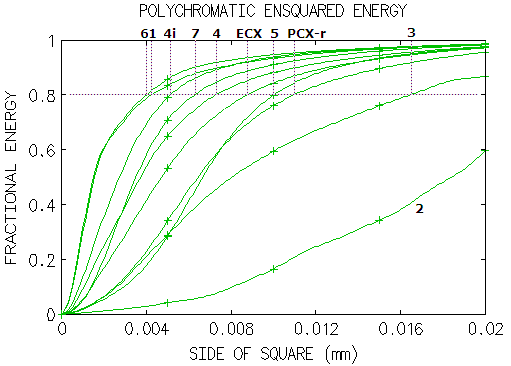Ensquared energy plots (400-800nm, typical CCD sensitivity) for each of these camera arrangements show that at 2
° off-axis PCX+PPP (6) packs up 80% of the energy (standard CCD spectral sensitivity in the 0.4-0.8 micron range) within 4μm square, slightly better than the stand alone camera over its best (curved) field (1), the integrated standard PCX (4i) within little over 5μm square, doublet flattener (7) little over 6μm, non integrated standard PCX (w/Schmidt corrector slightly closer to correct coma) little over 8μm square (4), ECX nearly 9μm, meniscus nearly 10μm square (5), and PCX-r (w/Schmidt corrector slightly closer to correct coma) little about 11μm. Standard PCX not corrected for residual coma (2) needs over 16μm square and, for comparison, stand alone camera over flat field about 23μm square. Since this is the field edge, probably all except 3 can be considered acceptable, with the 80% energy edge footprint of 0.01mm, or less. Table below shows the parameters for each of the above systems.

 CAMERA ALONE PCX STANDARD ECX PCX-r MENISCUS PCX+PPP DOUBLET Schmidt corrector A4 9.27E-10 9.27E-10 9.27E-10 9.27E-10 8.84E-10 8.15E-10 8.94E-10 A6 2.16E-15 2.16E-15 2.16E-15 2.16E-15 2.16E-15 2.16E-15 2.16E-15 RC -54,000 -54,000 -54,000 -54,000 -26,000 -140,000 -25,000 STOP-to-MIRROR 800 772* 777* 780* 790* 800 767* MIRROR-to-LENS - -394.5 -394.5 -394.5 -389.9 -378.5 -391.1 T-PPP - - - - - -17.2 (BK7) - Rl1 - -133 -296 inf. 660 -134 118 Tl1 - -3 (K11) -3 (K11) -3 (K11) -7.5 (K11) -3 (K11) -3.5 (BK7) Rl2 - inf. 296 -162 134 inf. 190 Tl2 - - - - - - -2.5 (F2) Rl3 - - - - - - inf. LENS-to-IMAGE - -1.92 -1.94 -1.96 -1.91 -1.9 -1.96

The asterisk is for corrector separation change needed to cancel coma. Corrector radius changes significantly with both, meniscus and PCX+PPP flatteners, which means that it shape also changes significantly. Since the radius is inversely proportional to the defocus parameter Λ, and for the stand-alone camera Λ=1 (0.707 neutral zone), the integrated meniscus flattener has Λ~2.1, i.e. Schmidt profile shape which would without flattener have brought all the wavelengths to a common focus slightly inside (toward mirror) marginal focus (convex profile with the neutral zone at 1.025, or slightly out of the effective surface). And the profile with the PCX+PPP flattener has Λ~0.386, i.e. neutral zone at 0.44 radius, with the profile edge higher than its inner portion. Both profiles are harder to fabricate than the standard 0.707 neutral zone.

Reducing lens-to-image separation would proportionally reduce the cone width at the lens, i.e. the effective aperture at the lens surfaces, and with it the level of aberrations. However, since the only significant aberration at the 2mm separation is coma, which can be cancelled by moving Schmidt corrector slightly closer, further reducing the lens-to-image separation would not produce practical benefits.

For the same reason - i.e. due to the smaller effective (cone) aperture at the lens - aberrations induced by the single-lens field-flattener will be much smaller in slow systems for any given lens-to-image separation: in proportion to the fourth power, third power and square of the inverse F-number for spherical aberration, coma and astigmatism, respectively. For that reason, monochromatic aberrations of a single-lens flattener are not the limiting factor to widening the lens-to-image separation in a system like Cassegrain. The limiting factors are lateral color, increasing approximately with the lens-to-image separation, and the magnification factor, since the flattener has negative power.

Doublets field flatteners

Two-lens subaperture corrector can flatten field in the Cassegrain or, in general, any of other popular two mirror systems. A long-focus Cassegrain gains less from field flattening than, for instance Ritchey-Chretien or Dall-Kirkham, both having stronger off-axis aberrations, which are also greatly reduced with adequately designed flattener.

Similarly, flattening field in the SCT with spherical mirrors would produce little benefit if not correcting for the system coma as well.

Example 2: SCT with spherical mirrors - A near zero-power pair of BK7 lenses for flattening the field of the commercial 9-inch ƒ/2/10 SCT. Coma is removed by the rear surface of the front lens, and the rear lens mainly produces net astigmatism that flattens the field. For the near-complete correction, a pair of lenses has to be an integral part of the system, i.e. Schmidt corrector adjustment is required. This flattener - somewhat tweaked version of the flattener/corrector for a ƒ/2.8/10 SCT by Richard Snashall - leaves a trace of residual coma, and induces near 1/4 wave (0.24) P-V of undercorrection. Either can be cancelled at the expense of the other becoming somewhat greater (for instance, zero coma would result in about 0.3 wave P-V of spherical aberration, and for negligible spherical - about 1/6 wave P-V or less - the RMS error would only slightly increase, but would have noticeably more of coma-like deformation toward the edge).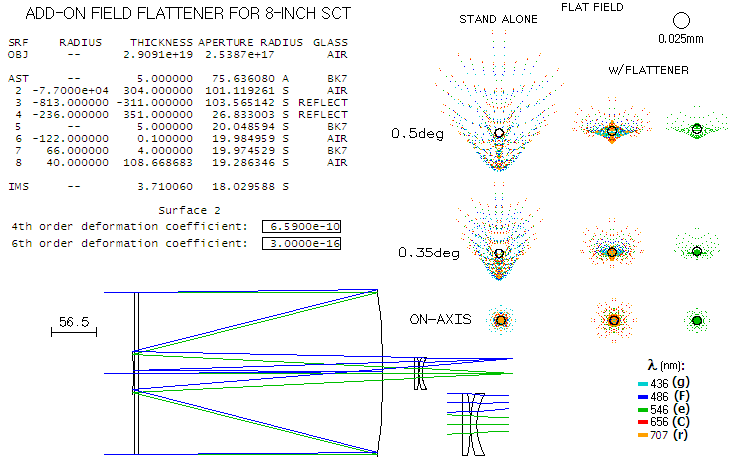A pair of simple plano CX/CV lenses can eliminate curvature of field in a Cassegrain without inducing significant chromatism, spherical aberration or coma. If the positive element is made of common crown, and the negative of common flint, the flattener also somewhat reduces the focal length, generally desirable with long-focus Cassegrains.

Example 3: Simple field flattener for a Cassegrain. 300mm ƒ/4/14 Classical Cassegrain with a flattener that improves its performance over wide field (there is no noticeable change in the blur size if the spectral range is widened). The flattener induces astigmatism which flattens the field. It also reduces the focal length by 7-8%. This particular flattener only illustrates the effect; somewhat better correction could be possible if optimized for the location, spacing and shape. But any further improvement wouldn't be significant in practical terms, since the dominant residual aberration is the field-flattening astigmatism.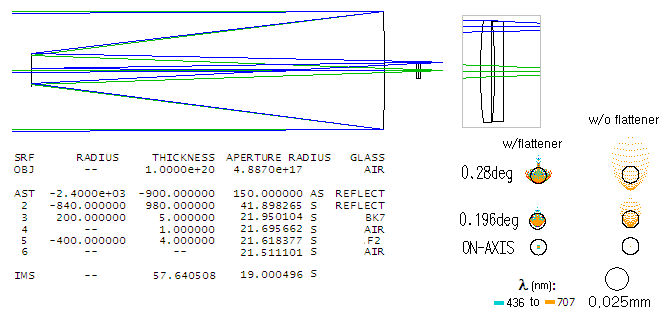Similar form of a field flattener can be used for refractors. Since most of them are fairly similar in their field curvature properties, the parameters of such flattener can be defined, at least in general terms, in terms of the refractor's focal length. A pair of plano CX/CV lenses of the common crown and flint (respectively) will induce field-flattening astigmatism and low residual aberrations if the crown lens has focal length of nearly 1/3 of the focal length ƒ of the refractor - which sets its radius at ~ƒ/3(n-1) - with the flint lens radius twice larger, and the two placed in near contact at about 0.1ƒ in front of the original focus. The astigmatism is nearly halved in magnitude, but becomes different in sign to somewhat stronger Petzval, with the net effect being near-flat field with roughly half the astigmatism of the objective lens alone.

Typically such flattener will reduce off axis error by a factor of 3. While it does flatten image field in both, achromats and apochromats, it is less beneficial in achromats, due to their large longitudinal chromatic defocus, as plots below illustrate. While the error is significantly reduced in the green, farther off-axis chromatic error with the flattener is larger than without it, because field curvature in part compensates for the chromatic defocus. With the red/green/blue blur being similar in size at 1° off axis, here would be little change in imaging, but visual field definition would be noticeably improved, since eye sensitivity is much higher for the green.

Example 4: Simple flattener for refractors - At left, farther off axis the green (e) blur in the achromat is noticeably smaller with flattener, but the red/blue (C/F) blur is actually larger, due to the curvature actually bringing C/F closer to focus. With CCD chip nearly as sensitive in the red/blue as in the green, there is little to gain in imaging. On the other hand, the gain is evident with apo refractors (note that the flattener reduces focal length by 10-15%).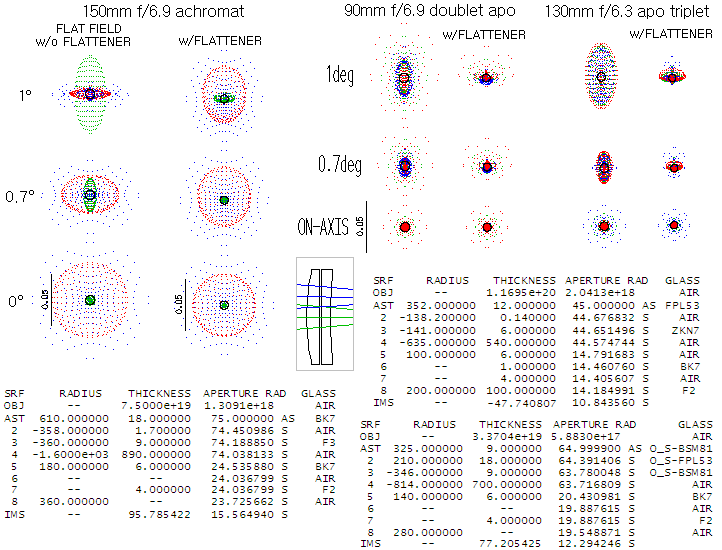With such a generic prescription, best location for the flattener varies from one system to another, but it should be within 10-20mm. At the optimum location, coma is near zero, and the median astigmatic surface is nearly flat (the error is actually slightly smaller with a very mild residual curvature, than with flat median surface, which requires more of astigmatism). Moving corrector away from the optimum location worsens the curvature/astigmatism error, and also induces coma (small changes in the lens spacing have little effect, the two lenses can be in full contact). Again, optimization would probably somewhat improve the best flattener's performance, put not significantly in practical terms, since the dominant aberration is the astigmatism flattening the field.

Example 5: Eyepiece flattener - Finally, for visuall use it is possible to flatten field of the objective with a zero-power singlet lens pair inserted into eyepiece barrel. Being very close to the image, the only significant aberration of such corrector is astigmatism, which can flatten the final visual field. Shown is such corrector that flattens field of a 150mm f/5 achromat, by inducing astigmatism of opposite sign to that of achromat's Petzval curvature. Final result is flat visual field and more than twice lower astigmatism.